## Name

ST_ChaikinSmoothing — Returns a smoothed version of a geometry, using the Chaikin algorithm

## Synopsis

`geometry ST_ChaikinSmoothing(`geometry geom, integer nIterations = 1, boolean preserveEndPoints = false`)`;

## Description

Smoothes a linear or polygonal geometry using Chaikin's algorithm. The degree of smoothing is controlled by the `nIterations` parameter. On each iteration, each interior vertex is replaced by two vertices located at 1/4 of the length of the line segments before and after the vertex. A reasonable degree of smoothing is provided by 3 iterations; the maximum is limited to 5.

If `preserveEndPoints` is true, the endpoints of Polygon rings are not smoothed. The endpoints of LineStrings are always preserved.The number of vertices doubles with each iteration, so the result geometry may have many more points than the input. To reduce the number of points use a simplification function on the result (see ST_Simplify, ST_SimplifyPreserveTopology and ST_SimplifyVW).

The result has interpolated values for the Z and M dimenions when present.This function supports 3d and will not drop the z-index.

Availability: 2.5.0

## Examples

Smoothing a triangle:

```SELECT ST_AsText(ST_ChaikinSmoothing(geom)) smoothed
FROM (SELECT  'POLYGON((0 0, 8 8, 0 16, 0 0))'::geometry geom) AS foo;

smoothed
───────────────────────────────────────────
POLYGON((2 2,6 6,6 10,2 14,0 12,0 4,2 2))
```

Smoothing a Polygon using 1, 2 and 3 iterations: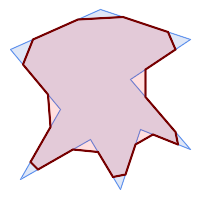nIterations = 1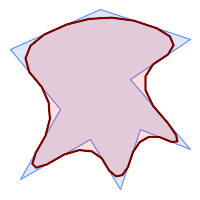nIterations = 2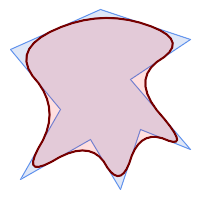nIterations = 3
```SELECT ST_ChaikinSmoothing(
'POLYGON ((20 20, 60 90, 10 150, 100 190, 190 160, 130 120, 190 50, 140 70, 120 10, 90 60, 20 20))',
generate_series(1, 3) );
```

Smoothing a LineString using 1, 2 and 3 iterations: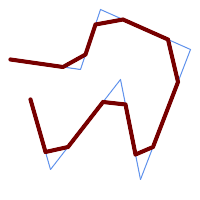nIterations = 1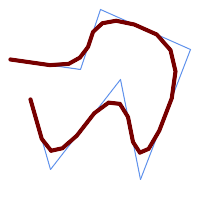nIterations = 2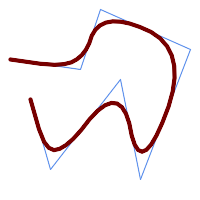nIterations = 3
```SELECT ST_ChaikinSmoothing(
'LINESTRING (10 140, 80 130, 100 190, 190 150, 140 20, 120 120, 50 30, 30 100)',
generate_series(1, 3) );
```